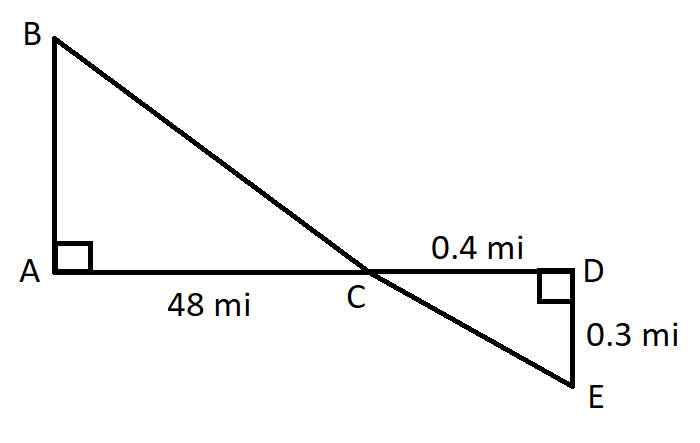Question# Use similar triangles to find the distance across the river.

Right triangles and trigonometry
ANSWEREDUse similar triangles to find the distance across the river.2021-02-10

$$\displaystyle∠{A}≅∠{D}$$ since all right triangles are congruent and $$\displaystyle∠{B}{C}{A}≅∠{E}{C}{D}$$ because vertical angle pairs are congruent. So, $$\displaystyle△{A}{B}{C}∼△{D}{E}{C}$$ by A.
Similar figures have corresponding side lengths that are proportional. If x is the distance across the river (which is BC), then we can write:
$$\displaystyle{B}\frac{{C}}{{E}}{C}={A}\frac{{C}}{{D}}{C}$$
$$\frac{x}{EC}=\frac{48}{0.4}$$
To find EC, use Pythagorean Theorem on $$\displaystyle△{D}{E}{C}$$:
$$\displaystyle{D}{E}^{{2}}+{D}{C}^{{2}}={E}{C}^{{2}}$$
$$\displaystyle{0.3}^{{2}}+{0.4}^{{2}}={E}{C}^{{2}}$$
$$\displaystyle{0.25}={E}{C}^{{2}}$$
0.5=EC
Hence,
$$\displaystyle\frac{{x}}{{0.5}}=\frac{{48}}{{0.4}}$$
$$\displaystyle\frac{{x}}{{0.5}}{\left({0.5}\right)}=\frac{{48}}{{0.4}}{\left({0.5}\right)}$$
x=60 mi2021-08-11